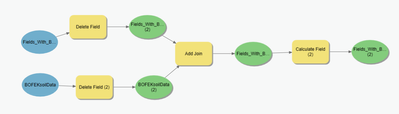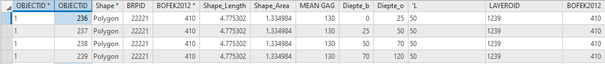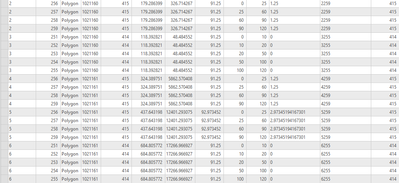# calculate field on a joined table with non unique OID

402
1
01-08-2021 05:18 AMNew Contributor II

Hello community,in the above picture I have joined a feature class with (agricultural) fields with a table with information on what type of soil layers are in those fields. the join worked great in that it gives x amount of layers based on the soil type per field.The first OBJECTID* is from the fields class and the second OBJECTID is from the layer table, both are not unique but combined they could be made unique (see below).

now I have to do certain calculations on it but I think because the OBJECTID's aren't unique it just takes one of the values, here is the code I used in the calculate field tool:

def Layerdepth(MEAN_GAG, Diepte_o, Diepte_b):
if (Diepte_o <= MEAN_GAG):
depth= Diepte_o-Diepte_b
return depth
elif (Diepte_o>=MEAN_GAG and Diepte_b<=MEAN_GAG):
depth= MEAN_GAG-Diepte_b
return depth
elif (Diepte_b>=MEAN_GAG):
return 0

I need to calculate the depth of each layer but now it just puts one depth at each layer as can be seen in the table above (depth='L).

because I think it is because the OBJECTID's aren't unique I tried creating unique OBJECTID's and then iterate over those. I used this expression for that:but then I run into the same problem that it puts the same value at each layer for each field as seen in table above.

Is there anyway I can adjust the code so it does calculate for each layer or a way to make a unique OID for each layer in each field so I can iterate over that?

Cheers,

Leendert